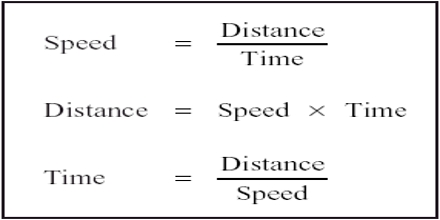Physics

# How to Calculate Speed?Prime purpose of this lecture is to present on How to Calculate Speed.  The speed of an object is how fast or slow it’s moving. You can calculate speed using the equation ‘speed = distance/time’. Distance-time graphs illustrate how an object moves. They show how the distance moved from a starting point changes over time. Units for speed Depends, but will always be a distance unit / a time unit. Example:  Cars: mi./h; Jets: km/h; Snails: cm/s; Falling objects: m/s etc.